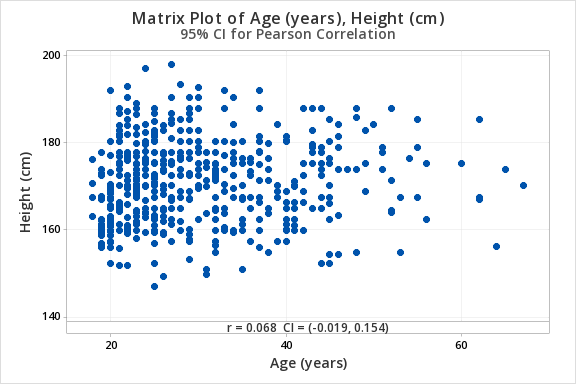# 12.2.1.2 - Example: Age & Height

12.2.1.2 - Example: Age & Height

Data concerning body measurements from 507 adults retrieved from body.dat.txt for more information see body.txt. In this example, we will use the variables of age (in years) and height (in centimeters) only.

For the full data set and descriptions see the original files:

For this example, you can use the following Minitab file: body.dat.mpx

Research question: Is there a relationship between age and height in adults?

1. Check assumptions and write hypotheses

Age (in years) and height (in centimeters) are both quantitative variables. From the scatterplot below we can see that the relationship is linear (or at least not non-linear).$$H_0: \rho = 0$$
$$H_a: \rho \neq 0$$

2. Calculate the test statistic

From Minitab:

##### Pairwise Pearson Correlations
Sample 1 Sample 2 N Correlation 95% CI for $$\rho$$ P-Value
Height (cm) Age (years) 507 0.068 (-0.019, 0.154) 0.127

$$r=0.068$$

3. Determine the p-value

$$p=.127$$

4. Make a decision

$$p > \alpha$$ therefore we fail to reject the null hypothesis.

5. State a "real world" conclusion.

There is not evidence of a relationship between age and height in the population from which this sample was drawn.

  Link ↥ Has Tooltip/Popover Toggleable Visibility